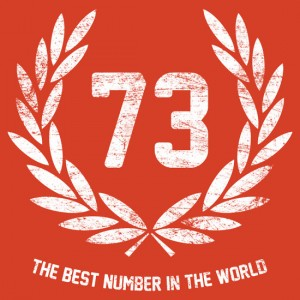# Why are Mathematicians obsessed with prime numbers?

Mathematics is a such a vast subject and still a lot of it has not been explored. Mathematicians are always looking for new riddles and puzzles to occupy them with. Which leads them to discover formulaes and numbers so complex that it can appear overwhelming to an average person.But most of the mathematicians believe that the solutions to the most of the complex problems lie in the basics. Just like Physicists are always trying to break down matter into simpler forms, so are the mathematicians. This invariably means a return to the counting numbers, 1, 2, 3, 4, 5, 6, 7, 8, 9, 10, 11, 12, . . . Can we get more basic than this?

One interesting fact about numbers is that some of them are breakable like 6=3×2, 4=2×2, and many more. Such numbers which can be broken down in a more primary form are called composite numbers. However numbers such as 2,5,3,7,11 can’t be broken down and are called primes. As a matter of fact every number in the number system can be represented as a product of primes. For example: 12=2x2x3, another number, 6,545,448 can be written, 23 × 35 × 7 × 13 × 37.

Studying prime numbers takes us back to the very basics of the basics. Prime numbers are important because they are the ‘atoms’ of mathematics. Like the basic chemical elements from which all other chemical compounds are derived, prime numbers can be built up to make mathematical compounds.

Now at this point I would like to share with you the ‘Best Number’:73. What is so fascinating and interesting about the number 73? Well for one it is the 21st prime number and its reverse 37 is the 12th prime number which is the reverse of 21. Not only this but the binary representation of 73, 1001001 is a palindrome as is the binary representation of 12, 10101. Such numbers whose reverse is also a prime number are called emirps. That is the reason scientists are also obsessed with the number 73.

If you want to know more about the number you can visit the link. http://en.wikipedia.org/wiki/73_(number)

Sexy Primes: In mathematics, sexy primes are prime numbers that differ from each other by six. For example, the numbers 5 and 11 are both sexy primes, because they differ by 6. If p + 2 or p + 4 (where p is the lower prime) is also prime, then the sexy prime is part of a prime triplet.

The term “sexy prime” stems from the Latin word for six: sex.

The number of the numerologist: Just like the previous example of the number 73 there are many more numbers which have uncanny properties making them popular among the mathematicians. But this number will probably the ahead than all others.

One of the most challenging areas of number theory concerns Waring’s problem. In this setting the magic arts of numerology meets the clinical science of mathematics in the shape of primes, sums of squares and sums of cubes. In numerology, take the unrivaled cult number 666, the ‘number of the beast’ in the biblical book of Revelation, and which has some unexpected properties. It is the sum of the squares of the first 7 primes:Just a quick 66 fact: The sum of the numbers on Russian roulette is 666.

666 = 22+ 32+ 52+ 72+ 112+ 132+ 172

Numerologists will also be keen to point out that it is the sum of palindromic cubes and, if that is not enough, the keystone 63 in the center is shorthand for 6 × 6 × 6:

666 = 13+ 23+ 33+ 43+ 53+ 63+ 53+43+ 33+ 23+ 13

The number 666 is truly the ‘number of the numerologist’.

We described the prime numbers as the ‘atoms of mathematics’. But ‘surely,’ you might say, ‘physicists have gone beyond atoms to even more fundamental units, like quarks. Has mathematics stood still?’ If we limit ourselves to the counting numbers, 5 is a prime number and will always be so. But Gauss made a far-reaching discovery, that for some primes, like 5, 5 = (1 – 2i) × (1 + 2i) where of the imaginary number system. As the product of two Gaussian integers, 5 and numbers like it are not as unbreakable as was once supposed.[wpedon id="7041" align="center"]